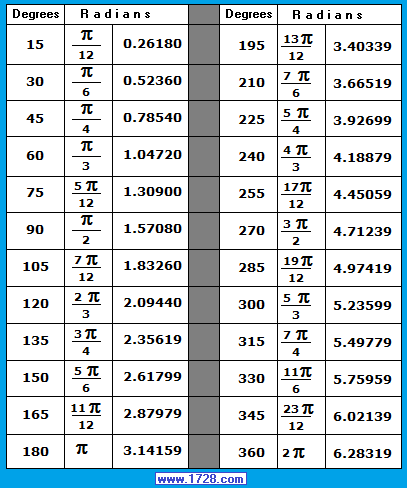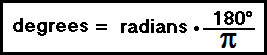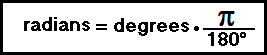Radians are another way in which angles are measured. For example, 45° may also be referred to as ¼π radians or .78540 radians. There are 2π radians in a circle or stated another way, 2π radians = 360°. So, 1 radian equals 360° ÷ 2 π = 57.2957795130823 degrees. To convert radians to degrees multiply by 57.2957795130823.Two radians equal how many degrees? degrees = 2 * radians * 180° ÷ π degrees = 2 * 57.296 = 114.59° To convert degrees to radians multiply by 0.0174532925199433.17 degrees equal how many radians? radians = 17 * π ÷ 180 radians = 17 * .017453 = 0.2967